Saturday, December 4, 2021
Home > CBSE Class 10 > Important MCQ Questions for Class 10 Maths Chapter 12 Area Related to Circles

# Important MCQ Questions for Class 10 Maths Chapter 12 Area Related to CirclesHi students, Welcome to AMBiPi (Amans Maths Blogs). In this article, you will get Important MCQ Questions for Class 10 Maths Chapter 12 Area Related to Circles. You can download this PDF and save it in your mobile device or laptop etc.

Important MCQ Questions of Area Related to Circles Class 10 Question No 21:

In the figure below, ABCDEF is a regular hexagon. If six circles of radius r are drawn taken vertices A, B, C, D, E and F as centres. What is the area of the shaded portion?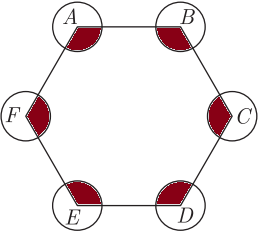Option A : πr2

Option B : 2πr2

Option C : 4πr2

Option D : 8πr2

Option B : 2πr2

Important MCQ Questions of Area Related to Circles Class 10 Question No 22:

In the figure below, if the side of the square is 4, then find the shaded area.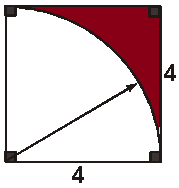Option A : (4 – π)

Option B : 2(4 – π)

Option C : 3(4 – π)

Option D : 4(4 – π)

Option D : 4(4 – π)

Important MCQ Questions of Area Related to Circles Class 10 Question No 23:

In the figure below, if MN = 2√2, then find the shaded area.Option A : 2π

Option B :

Option C :

Option D :

Option A :

Important MCQ Questions of Area Related to Circles Class 10 Question No 24:

In the figure below, three semicircles each of diameter 3 cm, a circle of diameter 4.5 cm and a semicircle of radius 4.5 cm are drawn in the given figure. The area of the shaded region will be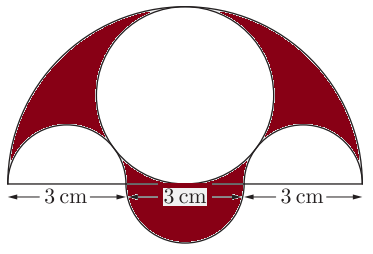Option A : 25.6 cm2

Option B : 50.6 cm2

Option C : 12.375 cm2

Option D : 28.375 cm2

Option C : 12.375 cm2

Important MCQ Questions of Area Related to Circles Class 10 Question No 25:

In the figure below, if AB = BC = 4, then find the shaded region.Option A : 4(4 – π)

Option B : 8(4 – π)

Option C : 12(4 – π)

Option D : 16(4 – π)

Option D : 16(4 – π)

Important MCQ Questions of Area Related to Circles Class 10 Question No 26:

In figure below, if AD = CD = L is the radius of sector of a circle with center as D, then find the shaded region.Option A : (3√3/4 – π/6)L2

Option B : (3√3/2 – π/6)L2

Option C : (3√3/8 – π/6)L2

Option D : (3√3/5 – π/6)L2

Option A : (3√3/4 – π/6)L2

Important MCQ Questions of Area Related to Circles Class 10 Question No 27:

In the figure below, ABDC is a quadrant of a circle of radius 28 cm and a semi-circle BEC is drawn with BC as diameter. What is the area of the shaded region?Option A : 392 cm2

Option B : 196 cm2

Option C : 208 cm2

Option D : 416 cm2

Option A : 392 cm2

Important MCQ Questions of Area Related to Circles Class 10 Question No 28:

In the figure below, if OA = OB = 4, then find the area of the shaded region.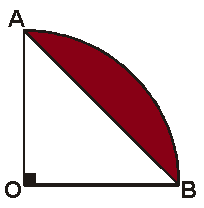Option A : 2(π – 2)

Option B : 3(π – 2)

Option C : 4(π – 2)

Option D : 5(π – 2)

Option A : 2(π – 2)

Important MCQ Questions of Area Related to Circles Class 10 Question No 29:

In the figure below, ABCD is a square of side 10 units and two quadrant of the circles are drawn inside the square. Find the shaded area.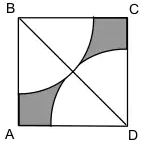Option A : 25(3 – 2π)

Option B : 25(2 – π)

Option C : 25(4 – 3π)

Option D : 25(4 – π)

Option D : 25(4 – π)

Important MCQ Questions of Area Related to Circles Class 10 Question No 30:

The long and short hands of a clock are 6 cm and 4 cm long respectively. What is the sum of distances travelled by their tips in 24 hours. (nearest integer)

Option A : 810

Option B : 955

Option C : 620

Option D : 710

Option B : 955

CBSE Class 10 Maths Chapterwise MCQs
1 : Real Numbers MCQ
| 1 to 10 | 11 to 20 | 21 to 30 | 31 to 40 | 41 to 50
2 : Polynomials MCQ
| 1 to 10 | 11 to 20 | 21 to 30 | 31 to 40 | 41 to 50
3 : Linear Equations MCQ
| 1 to 10 | 11 to 20 | 21 to 30 | 31 to 40 | 41 to 50
| 1 to 10 | 11 to 20 | 21 to 30 | 31 to 40 | 41 to 50
5 : Arithmetic Progression MCQ
| 1 to 10 | 11 to 20 | 21 to 30 | 31 to 40 | 41 to 50
6 : Triangles MCQ
| 1 to 10 | 11 to 20 | 21 to 30 | 31 to 40 | 41 to 50
7 : Coordinate Geometry MCQ
| 1 to 10 | 11 to 20 | 21 to 30 | 31 to 40 | 41 to 50
8 : Introduction to Trigonometry MCQ
| 1 to 10 | 11 to 20 | 21 to 30 | 31 to 40 | 41 to 50
9 : Application of Trigonometry MCQ
| 1 to 10 | 11 to 20 | 21 to 30 | 31 to 40 | 41 to 50
10 : Circles MCQ
| 1 to 10 | 11 to 20 | 21 to 30 | 31 to 40 | 41 to 50
11 : Constructions MCQ
| 1 to 10 | 11 to 20
12 : Area Related to Circles MCQ
| 1 to 10 | 11 to 20 | 21 to 30 | 31 to 40 | 41 to 50
13 : Surface Area & Volume MCQ
| 1 to 10 | 11 to 20 | 21 to 30 | 31 to 40 | 41 to 50
14 : Statistics MCQ
| 1 to 10 | 11 to 20 | 21 to 30 | 31 to 40 | 41 to 50
15 : Probability MCQ
| 1 to 10 | 11 to 20 | 21 to 30 | 31 to 40 | 41 to 50
16 : Previous Year Maths MCQs from CBSE Board Class 10
| 1 to 100
AMBiPi
error: Content is protected !!